...Blending Modes

Introduction

Blending modes allow you to adjust how the colors in one layer mix with the colors in the layers below it. Most graphics programs offer a set of blending modes, but the number, naming and to some extent, the operation of these vary from program to program. This article introduces the set of blending modes implemented by Chasys Draw IES and shows the reader how to use these to achieve various effects.

Blending Modes

The two images below are the samples used in demonstrating Chasys Draw IES blending modes in the listing that follows. Note that both images have transparent and translucent parts to demonstrate how various blend modes interact with transparency.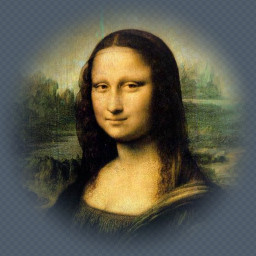Background Layer with Mode

Currently, Chasys Draw IES Artist supports the twenty-eight (28) blend modes listed below. The last mode, custom, can be used to extend the number of supported modes indefinitely.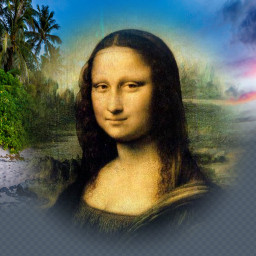Normal (Alpha Blend)

Uses the alpha of the current layer to mix it with the content below it.

Output = Blend (A, B, f ) = { A * ( 1- f ) } + ( B * f )None

Applies the current layer as is to the content below it, replacing all pixels without an form of blending.

Output = BMask

Applies the alpha of the current layer to the content below it.

Output = A + Alpha (B)Luma Mask

Converts the luma of the current layer into an alpha mask, combines it with the existing alpha then applies it to the content below.

Output = A + MakeAlpha { Luma (B) + Alpha (B) }Behind

Uses the pixels in the current layer to replace only the transparent pixels in the content below it, giving the impression that the current layer is behind the content.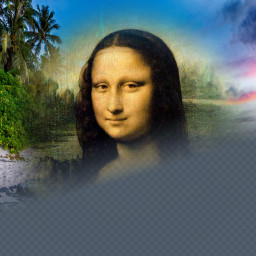Overpaint

Uses the pixels in the current layer to replace only the opaque pixels in the content below it, giving the impression that the current layer is behind the contentAdds the values in the current layer to the content below it, weighted by the alpha value.

Output = Blend (A, { B + Alpha(A) }, f )Add

Adds the values in the current layer to the content below it, weighted by the alpha value.

Output = Blend (A, { A+B }, f )Subtract

Subtracts the values in the current layer from the content below it, weighted by the alpha value.

Output = Blend (A, { A-B }, f )Multiply

Uses the values in the current layer to multiply the values in the content below it, weighted by the alpha value.

Output = Blend (A, { A*B }, f )Divide

Uses the values in the current layer to divide the values in the content below it, weighted by the alpha value.

Output = Blend (A, { A/B }, f )Offset

Adds the deviation of values in the current layer from the mid value to the content below it, weighted by the alpha value.

Output = Blend (A, { A + (B-0.5) }, f )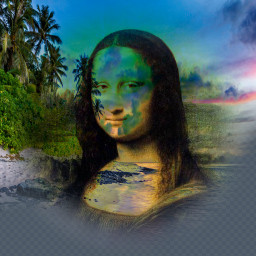Minimum

Picks the lowest corresponding values between those in the current layer and the content below it, weighted by the alpha value.

Output = Blend (A, Min(A,B), f )Maximum

Picks the highest corresponding values between those in the current layer and the content below it, weighted by the alpha value.

Output = Blend (A, Max(A,B), f )Difference

Picks the absolute difference between corresponding values in the current layer and the content below it, weighted by the alpha value.

Output = Blend (A, Abs(A-B), f )Screen

Blends in the inverse of multiplying the values in the current layer with the content below it.

Output = Blend (A, { 1 - (A*B) }, f )Overlay

Combines Multiply and Screen blend modes. The parts of the top layer where base layer is light become lighter, the parts where the base layer is dark become darker. An overlay with the same picture looks like an S-curve.

Output = Blend (A, {
 2*A*B , if A<0.5 1 - {2*(1-A)*(1-B)} , otherwise
}   , f )Dodge

Blends in the result of dividing the existing content by the inverted top layer. This lightens the bottom layer depending on the value of the top layer.

Output = Blend (A, { A/(1 - B) }, f )Burn

Blends in the inverse of dividing the inverted existing content by the top layer. This darkens the top layer, increasing the contrast to reflect the color of the existing content.

Output = Blend (A, { 1 - { (1 - A)/B } }, f )Luma

Applies the luminance of the current layer to the content below it, weighted by the alpha value.

Output = Blend (A, { Chroma (A) | Luma (B) }, f )Chroma

Applies the chromaticity of the current layer to the content below it, weighted by the alpha value.

Output = Blend (A, { Chroma (B) | Luma (A) }, f )Hue

Applies the hue of the current layer to the content below it, weighted by the alpha value.

Output = Blend (A, { Hue (B) | Saturation (A) | Value (A) }, f )Saturation

Applies the saturation of the current layer to the content below it, weighted by the alpha value.

Output = Blend (A, { Hue (A) | Saturation (B) | Value (A) }, f )Value

Applies the HSV value of the current layer to the content below it, weighted by the alpha value.

Output = Blend (A, { Hue (A) | Saturation (A) | Value (B) }, f )Lightness

Applies the HSL lightness of the current layer to the content below it, weighted by the alpha value.

Output = Blend (A, { Hue (A) | Saturation (A) | Lightness (B) }, f )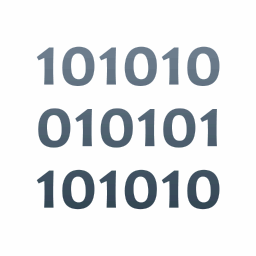Logical AND

Applies a logical (binary/bitwise) “AND” operation of the current layer to the content below it, weighted by the alpha value.

Output = Blend (A, { A and B }, f )Logical OR

Applies a logical (binary/bitwise) “OR” operation of the current layer to the content below it, weighted by the alpha value.

Output = Blend (A, { A or B }, f )Logical XOR

Applies a logical (binary/bitwise) “XOR” operation of the current layer to the content below it, weighted by the alpha value.

Output = Blend (A, { A xor B }, f )Custom

Applies a user-defined or plug-in-defined blend-mode based on the contents of a BLND attachment. Custom is normally used by plug-ins to render blend-modes that are not supported natively by Chasys Draw IES, e.g. Photoshop’s “Soft Light” mode.

Output = Blend (A, { Calculate (metadata.BLND, A, B) }, f )

Chasys Draw IES comes pre-packaged with some unique blending modes from Photoshop, GIMP and Paint.NET in the form of BLND attachment files that Chasys Draw IES can load for you when you select this image mode.